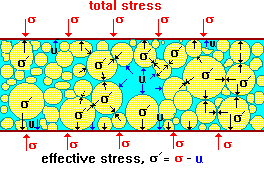Feb 26, 2016

Feb 26, 2016

Feb 26, 2016

# Methods to Determine Stress in SoilBoth immediate and consolidation settlement analysis requires estimate of increase in pressure (ΔHσ) in the soil layers from the applied loads. Several methods are available to estimate the increase in pressure at any depth z from the applied load. We will discuss:

1. 2:1 Slope method
2. Boussinesq Method.

## 2h: 1v Slope Method

An early method is to use 2 (horizontal): 1 (vertical) slope as shown in figure. For rectangular footing (B x L), the vertical pressure increase ΔHσ(z) at a depth z beneath the loaded area due to base load P is:

For square footing the above equation simplifies toThe 2:1 method compares reasonably well with more theoretical methods from z1 = B to about z2 = 4B but should not be used in the depth zone from z = 0 to B. The average stress increase in a stratum from H = Z2 – Z1 is

Also See: GeoTechnical Engineering

## Boussinesq Method of Stress Determination:

This method is based on theory of elasticity and is basically developed for a semi-infinite, isotropic, homogeneous half-space, however the method is used for all type of masses (layered etc), Newmark presented numerical solution to the Boussinesq equation, which is applicable beneath the corner of an area B x L.

Δσ = q0 m Iα

Where m is the number of corners contributing to is Δσ; m = 1 when Δσ under the corner of a footing is required and m=4 if Δσ under the center of a footing is required.